• 已知屏面上不重合的三点，如何求平面法向量？ 已知平面上不重合的三点o(0,0,0)，a(a1,a2,a3)，b(b1,b2,b3)，那么此三点所确定平面的法向量n(x,y,z)中的x,y,z值分别是多少？谢谢！ 一个平面应该有两个方向上...


已知屏面上不重合的三点，如何求平面法向量？

已知平面上不重合的三点o(0,0,0)，a(a1,a2,a3)，b(b1,b2,b3)，那么此三点所确定平面的法向量n(x,y,z)中的x,y,z值分别是多少？谢谢！
一个平面应该有两个方向上的法向量，Aireds所给出的答案法向量是指向哪个方向的？

用定义做

任取其中两点成为一条直线
oa向量(a1,a2,a3) ob向量(b1,b2,b3)
(x,y,z)*(a1,a2,a3)=0
(x,y,z)*(b1,b2,b3)=0
解得((a2b3-a3b2)/(a3b1-a1b3)y,y,(a2b1-a1b2)/(a1b3-b1a3)y)
把y消掉通分得(a2b3-a3b2,a3b1-a1b3,a1b2-a2b1)
x=a2b3-a3b2,y=a3b1-a1b3,z=a1b2-a2b1


展开全文• ## OpenGl法向量计算

千次阅读 2015-04-08 15:24:37
如何计算顶点法向量？ 三、我的总结 网上对于法向量的计算方法有多种：1.顶点周围三角面片的法向量的平均值 2.加权算法 3.....等，大同小异，无法就是个近似值，每种方法在不同的情景中，所得的近似值各有千秋。...
一、简介
顶点法向量的作用： 对渲染时光照的影响，造成不同的反射角度。
法向量：垂直于顶点所在的最小平面的单位向量，这个值是近似值。
二、目的
如何计算顶点法向量？
三、我的总结
网上对于法向量的计算方法有多种：1.取顶点周围三角面片的法向量的平均值 2.加权算法 3.....等，大同小异，无法就是取个近似值，每种方法在不同的情景中，所得的近似值各有千秋。
本文参考外文代码，给出实现的demo。
先参看下图;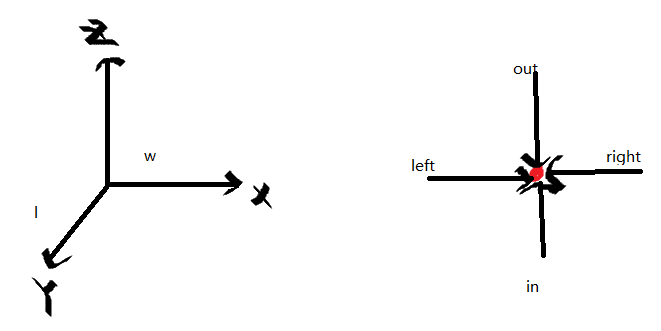代码是个加载地形的类：

class Terrain{
private:
int w;
int l;
float** hs;
Vec3f** normals;
bool computedNormals;
public:
Terrain(int w2, int l2){
w = w2;
l = l2;

hs = new float*[l];
for (int i = 0; i < l; i++){
hs[i] = new float[w];
}

normals = new Vec3f*[l];
for (int i = 0; i < l; i++){
normals[i] = new Vec3f[w];
}

computedNormals = false;
}

~Terrain(){
for (int i = 0; i < l; i++){
delete [] hs[i];
}
delete[] hs;

for(int i = 0; i < l; i++){
delete[] normals[i];
}
delete[] normals;
}

int width(){
return w;
}

int length(){
return l;
}

void setHeight(int x, int z, float y){
hs[z][x] = y;
computedNormals = false;
}

float getHeight(int x, int z){
return hs[z][x];
}

void computeNormals(){
if(computedNormals){
return;
}

Vec3f** normals2 = new Vec3f*[l];
for (int i = 0; i < l; i++) {
normals2[i] = new Vec3f[w];
}

for(int z = 0; z < l; z++){
for (int x = 0; x < w; x++){
Vec3f sum(0.0f, 0.0f, 0.0f);

Vec3f out;
if (z > 0)
{
out = Vec3f(0.0f, hs[z-1][x] - hs[z][x], -1.0f);
}

Vec3f in;
if(z < l - 1){
in = Vec3f(0.0f, hs[z + 1][x] - hs[z][x], 1.0f);
}

Vec3f left;
if (x > 0){
left = Vec3f(-1.0f, hs[z][x-1] - hs[z][x], 0.0f);
}

Vec3f right;
if (x < w - 1){
right = Vec3f(1.0f, hs[z][x+1] - hs[z][x], 0.0f);
}

if (x > 0 && z > 0){
sum += out.cross(left).normalize();
}

if (x > 0 && z < l - 1){
sum += left.cross(in).normalize();
}

if(x < w - 1 && z < l - 1){
sum += in.cross(right).normalize();
}

if (x < w - 1 && z > 0){
sum += right.cross(out).normalize();
}

normals2[z][x] = sum;
}
}
const float FALLOUT_RATIO = 0.5f;
for (int z = 0; z < l; z++)	{
for(int x = 0; x < w; x++){
Vec3f sum = normals2[z][x];

if(x > 0){
sum += normals2[z][x-1] * FALLOUT_RATIO;
}

if(x < w - 1){
sum += normals2[z][x+1] * FALLOUT_RATIO;
}

if(z > 0){
sum += normals2[z-1][x] * FALLOUT_RATIO;
}

if(z < l -1){
sum += normals2[z+1][x] * FALLOUT_RATIO;
}

if(sum.magnitude() == 0){
sum = Vec3f(0.0f, 1.0f, 0.0f);
}

normals[z][x] = sum;
}
}

for (int i = 0; i < l; i++)
{
delete[] normals2[i];
}
delete[] normals2;

computedNormals = true;
}

Vec3f getNormal(int x, int z){
if (!computedNormals){
computeNormals();
}

return normals[z][x];
}
};

函数void computeNormals()便是计算法向量方法。


展开全文• ## Meshlab计算点云法向量求三角网格

千次阅读 热门讨论 2018-05-27 17:20:17
最近在看曲面重建，感觉... 今天主要给大家演示一下如何对点云obj模型进行法向量的求和曲面重建。1.在meshlab中导入一个obj模型，此模型是我重采样后导出的模型（没显示特征点），所以除了点信息（V），任何...
        最近在看曲面重建，感觉meshlab的功能真的很强大，这也是为什么之前我要编译源码，想看源代码的原因之一。可参考我之前写的文章  Meshlab源码编译 VS2013+QT5.8.0。        今天主要给大家演示一下如何对点云obj模型进行法向量的求取和曲面重建。1.在meshlab中导入一个obj模型，此模型是我重采样后导出的模型（没显示特征点），所以除了点信息（v），任何信息都没有。如下图可以看到这个模型有3371个点，但是没有面信息。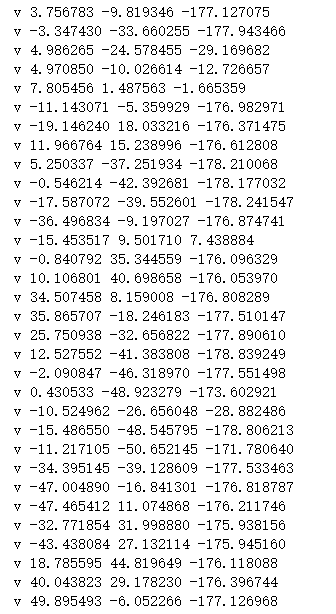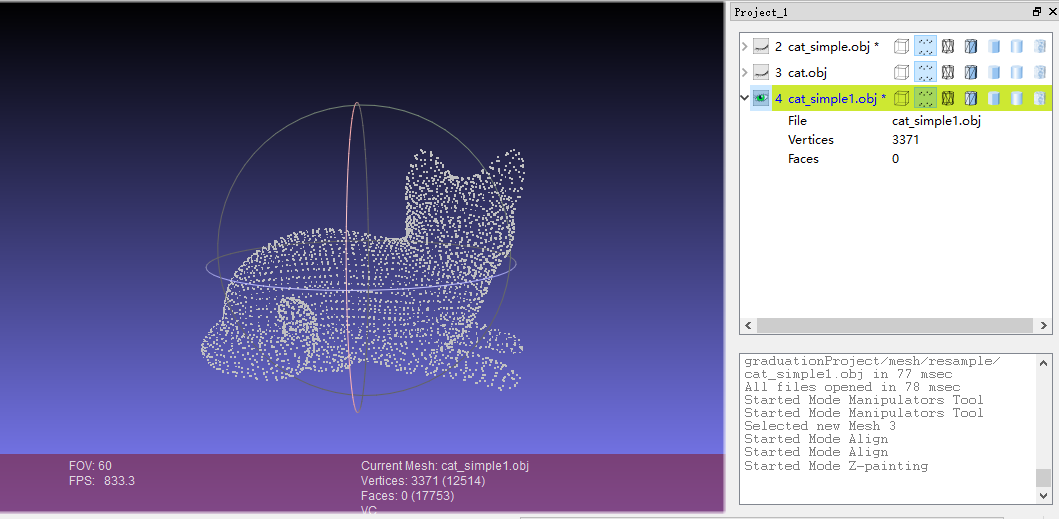2.求这个cat.obj模型的法向量，按下图选择蓝色选项框中的选项，会显示一个对话框，一般可以用默认值，近邻数也可以自己选择填写，之后选择Apply。（这里面有还有一个求法向量的方法，是Compute normals for point sets，效果一样）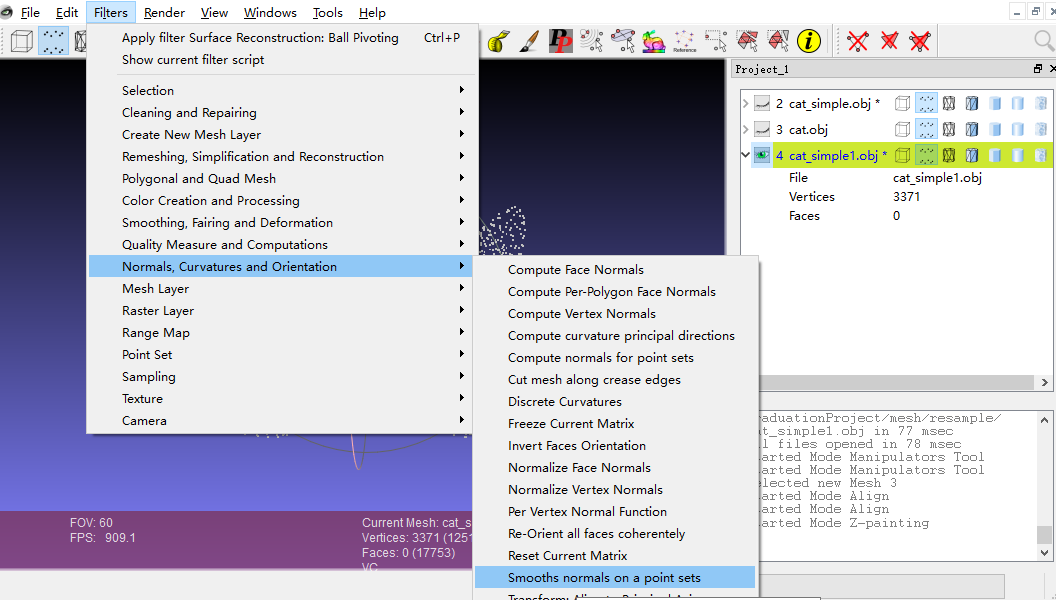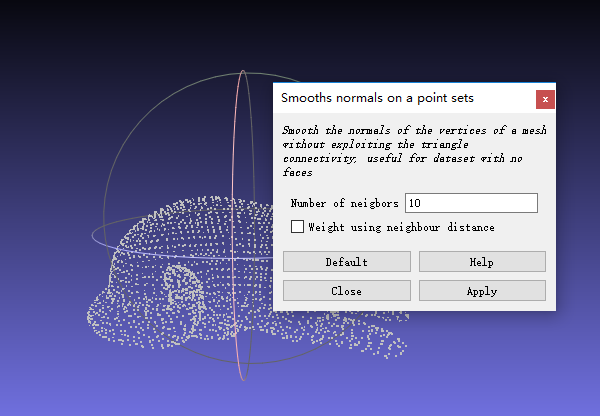3.对模型进行曲面重建，按下图选择蓝色选项框中的选项，球旋转的方法（因为我用了泊松重建和VCG的方法，感觉重建出来的效果不好）。弹出一个对话框，先用默认的参数点击Apply，可以看到面（Faces）增加到6262，看到效果一，并不是很好，还有漏洞，说明参数有点问题，当我把球半径加到23多的时候，面信息加到6662，此时模型网格化成功，曲面重建也成功了。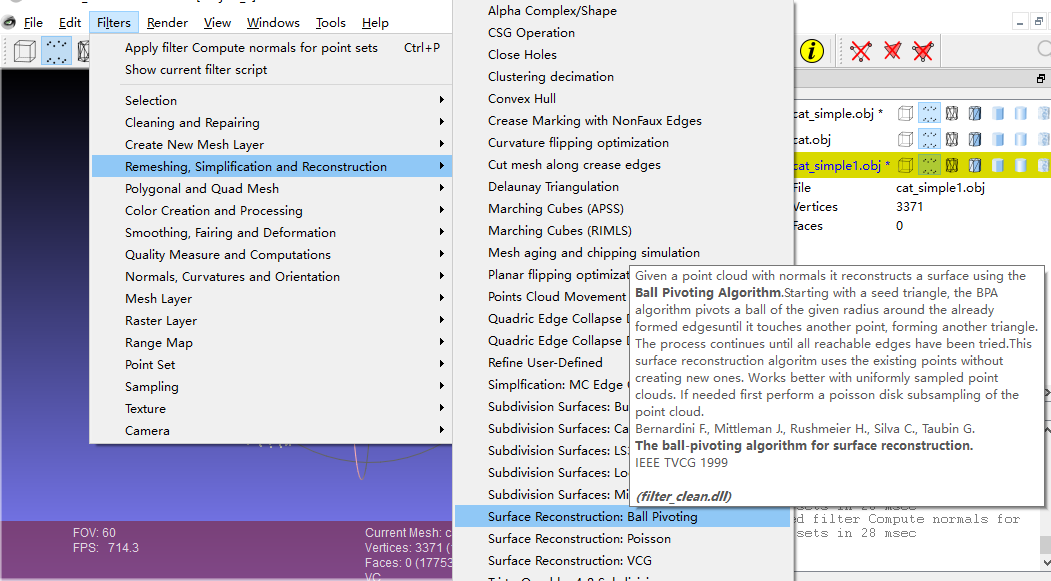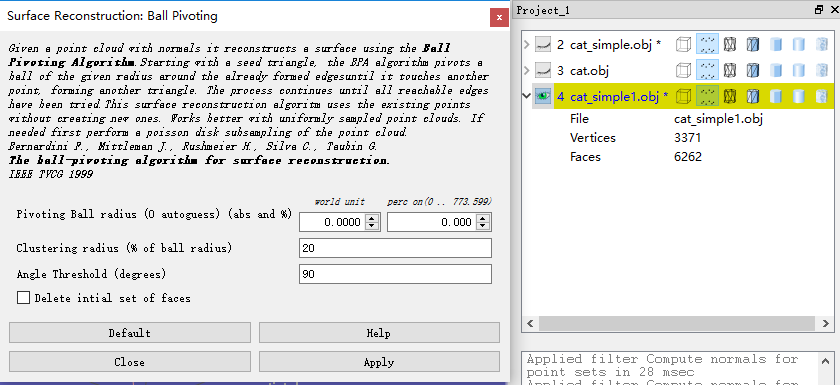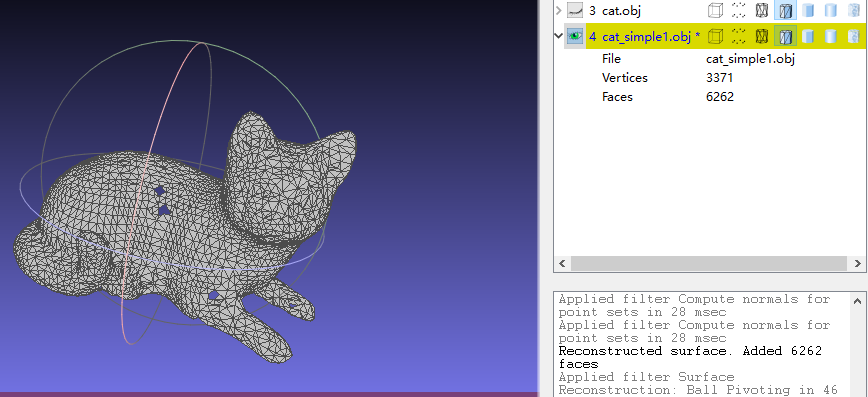效果一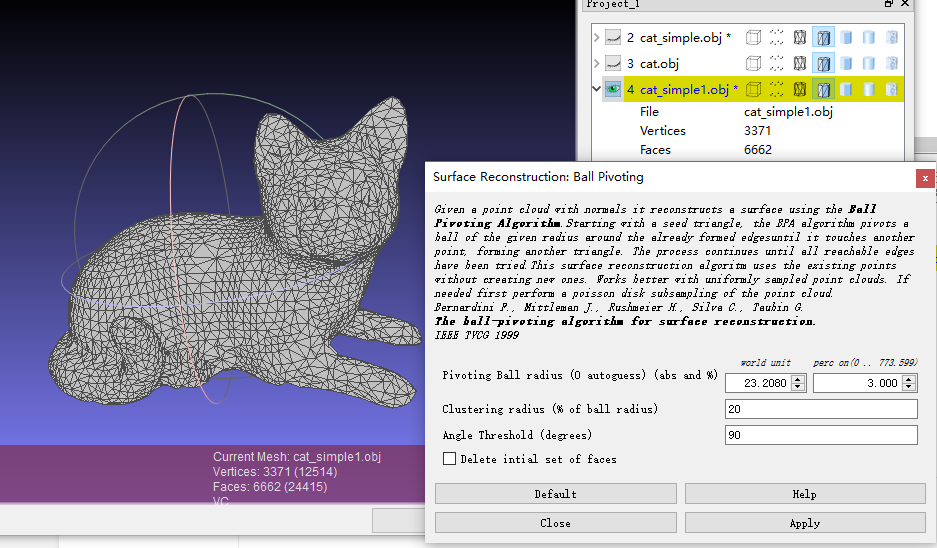效果二4.对模型进行保存，File->Export Mesh As,然后填写你要保存的文件名和类型，点save，把法向量（Normal）信息保存下来即可。打开刚才保存的文件，可以看到不仅有点信息（v），还有法向量信息（vn）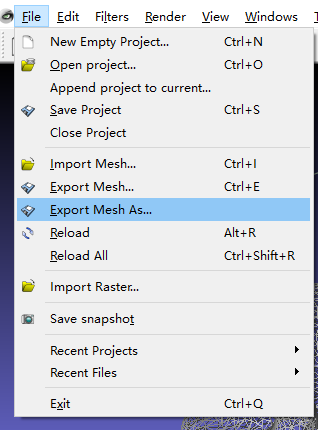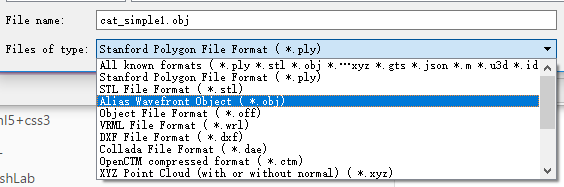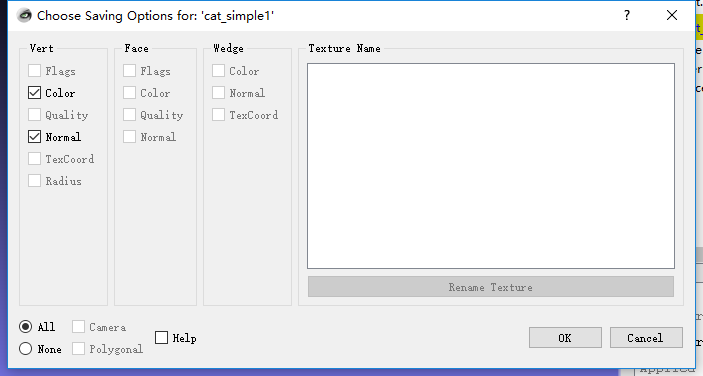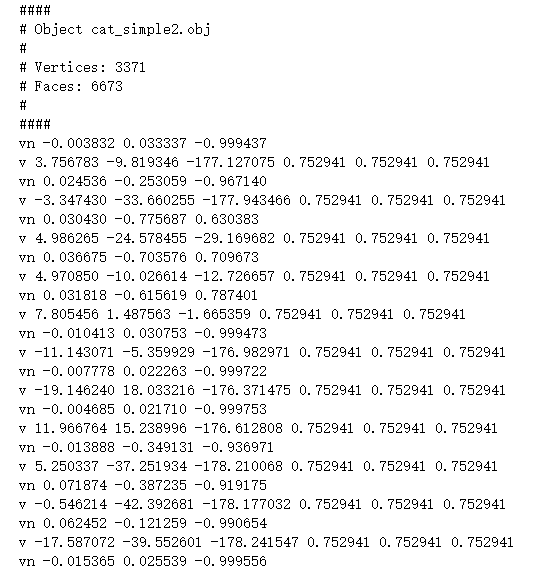展开全文• 在图形学中，计算光照模型时，经常需要求反射向量，一般的shader函数库都提供计算反射向量的方法，下面介绍一下如何手动计算反射向量。 给定入射光线向量I和平面法向量N，求反射向量R，如下图。为了方便计算，...
求反射向量

在图形学中，计算光照模型时，经常需要求取反射向量，一般的shader函数库都提供计算反射向量的方法，下面介绍一下如何手动计算反射向量。
给定入射光线向量I和平面法向量N，求反射向量R，如下图。为了方便计算，这里假定I和N都是单位向量（模为1，编程时可先将I和N单位化）
方法一设入射光线向量I和反射平面的法向量N之间的夹角为theta。连接I的始端和R的末端，则有
R = 2P - I              （1）
现在问题变成了如何求取P，设入射点0到P与N的交点的向量为S，那么有
P = I + S               （2）
现在问题变成了如何求取向量S，向量S即向量-N（注意，这里是-N，因为S和N的方向相反。）在向量N上的投影，根据向量的投影公式有因为N是单位向量，简化一下得到将S代入公式（2），再将P代入公式（1）得到方法二
将R平移一下，与向量N的延长线相交。由于入射角和反射角相等，且I和R的长度也相等，所以三角形ION是等腰三角形。故有
ON = 2S
所以有
R = I + 2S
而S是-I在N上的投影，所以有由于N是单位向量，简化一下得到所以貌似方法二更直观些。

向量投影
给定一个向量u和v，求u在v上的投影向量，如下图。假设u在v上的投影向量是u’，且向量u和v的夹角为theta。一个向量有两个属性，大小和方向，我们先确定u’的大小（即长度，或者模），从u的末端做v的垂线，那么d就是u’的长度。而u’和v的方向是相同的，v的方向v/|v|也就是u’的方向。所以有(1)
再求d的长度。(2)
最后求cos(theta)(3)
联合求解方程（1）（2）（3）得到这就是最终的投影向量。
而这个向量的长度d是============================
以下是旧的推导，也保留。转载于:https://www.cnblogs.com/mazhenyu/p/6700268.html
展开全文• 今天在工作中碰到一个问题，已知一个入射单位向量和发现向量如何求出反射单位向量，看到篇文章写...给定入射光线向量I和平面法向量N，求反射向量R，如下图。为了方便计算，这里假定I和N都是单位向量（模为1，编程时...
• 在图形学中，计算光照模型时，经常需要求反射向量，一般的shader函数库都提供计算反射向量的方法，下面介绍一下如何手动计算反射向量。 给定入射光线向量I和平面法向量N，求反射向量R，如下图。为了方便计算，这里...
• 在图形学中，计算光照模型时，经常需要求反射向量，一般的shader函数库都提供计算反射向量的方法，下面介绍一下如何手动计算反射向量。 给定入射光线向量I和平面法向量N，求反射向量R，如下图。为了方便计算，这里...
• 在图形学中，计算光照模型时，经常需要求反射向量，一般的shader函数库都提供计算反射向量的方法，下面介绍一下如何手动计算反射向量。 给定入射光线向量I和平面法向量N，求反射向量R，如下图。为了方便计算，...
• 在图形学中，计算光照模型时，经常需要求反射向量，一般的shader函数库都提供计算反射向量的方法，下面介绍一下如何手动计算反射向量。 给定入射光线向量I和平面法向量N，求反射向量R，如下图。为了方便计算，...Reflect
• 如何理解拉格朗日乘子和KKT条件,可以看这篇文章 拉格朗日乘子的简单介绍 拉格朗日乘子的具体介绍可以看上面的文章,我这边就简单的说一下它的核心 概念介绍 首先简单的讲一下梯度 方向导数是各个方向上的导数 ...机器学习 算法
• 线积分： 基于二重积分和三重积分的引入，我们对于线积分的引入过程将会轻车熟路。 对于一根不均匀密度的... 这里我们将这段曲线分割成n个区间段，可以近似求解质量，而随着n趋向无穷，这种近似的取法最终将...
• 几种取法种数汇总 无序有放回取法 假设可取的菜品有3种编号...可以明白每一个向量代表的就是一种情况且唯一，向量的个数就是可能的取法种类数。 于是可以得到以下等式： 其中，x1 x2 x3表示对应菜品被选中的次数，x
• <code>clc clear all close all %% 常数定义 M=15; %阵元数 snapshots=...floor(35e6/fs*NFFT):ceil(45e6/fs*NFFT) % 100个频点&...如何用波束形成做宽带信号DOA估计 <p>RfXX是无穷矩阵 如何解决</p>c语言 有问必答...